# Description

The CalcGen Generator calculates the result of an equation using up to five variables. Variables can either be constant values or references to other Attributes.

Example Equations:

• var1 + var2
• (var2 / 15) - var3 + 3 * (var1)
• (var4 * var3)  / var5 - var2 + var1

Example Variables:

• 3
• #{test.value1}

## Generator Parameters

The following parameters may be configured for the CalcGen Generator. Items with an asterisk* are required.

• equation* - The math equation to be evaluated.
• numberFormat* - Defines how the generated number will be formatted. Examples: 0, 0.00. #.00, #,##.00, \$#,##0.00.
• var1 - The first of five possible variables to use in the equation.
• var2 - The second of five possible variables to use in the equation.
• var3 - The third of five possible variables to use in the equation.
• var4 - The fourth of five possible variables to use in the equation.
• var5 - The fifth of five possible variables to use in the equation.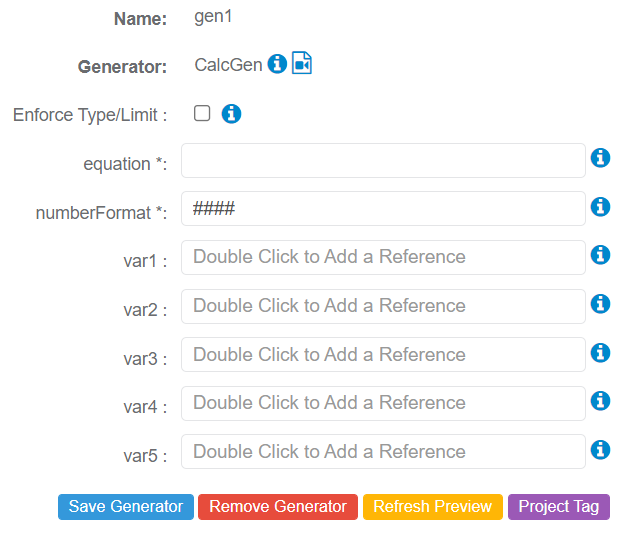## Special Note - Generating Output as Double Value or Float Value

The user must include d in the equation parameter after any/both of the var values for Double Value. An f should be used for float values. This is to specify the value of the var value is either a Double or Float Value.

• f - up to 7 digits
• d - up to 15 digits

Note: For 'd', the number format is ###0.00000000000000. The number of zeros after the decimal can be reduced if desired.

Example 1 - "f" Not Added to Var Values in Equation

equation = (var1 / var2) * 2

numberFormat = ####.000

var1 = 250

var2 = 60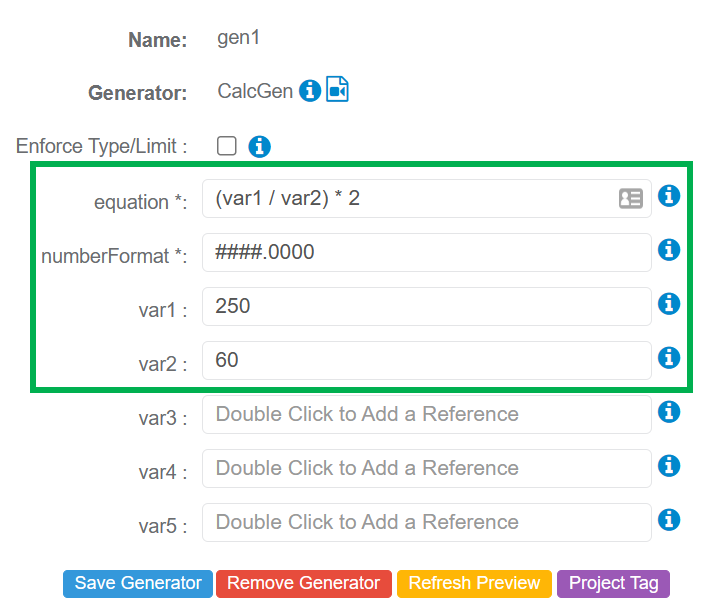Output is not in FLOAT Format. The correct value should be 8.3333, not 8.0000.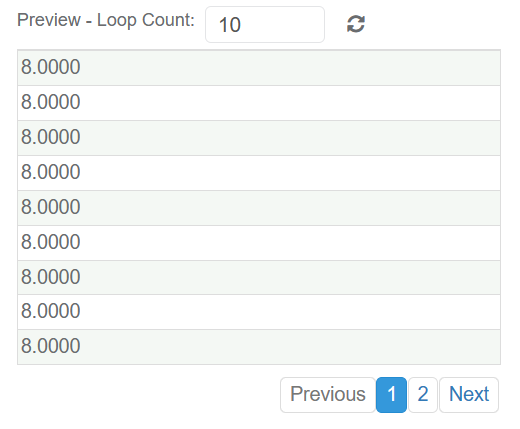Example 2 - "f" Added to Var2 in Equation

equation = (var1 / var2f) * 2

numberFormat = ####.000

var1 = 250

var2 = 60Output is now in FLOAT format and shows the correct value: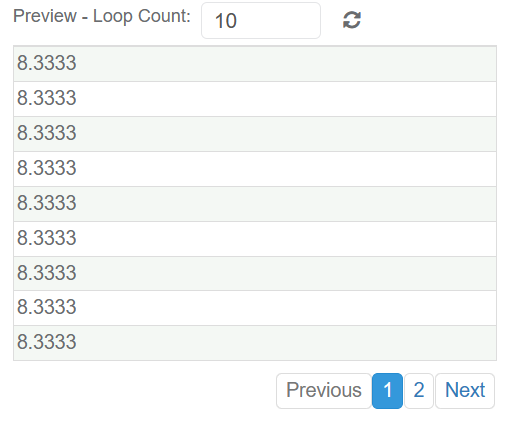## Example 1 - Calculate Regular, Overtime, and Total Pay Using an Equation

For this example, five Attributes have been added to calculate the regular pay, overtime pay, and total pay for individuals.

• fullName - Generates the full name of a person.
• hours - Generates work hours ranging from 1 to 80.
• regularPay - Generates regular pay based on the number of hours worked.
• overtimePay - Generates overtime pay based o the number of hours worked.
• totalPay - Adds the values generated for regular pay and overtime pay.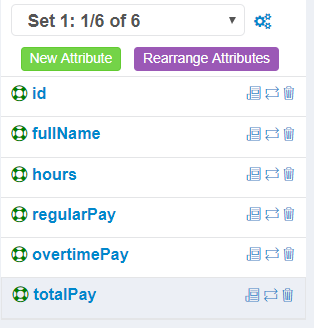### hours Attribute - Generator Configuration

The RandomGen Generator has been configured for the hours Attribute to generate the number of work hours between 1 and 80.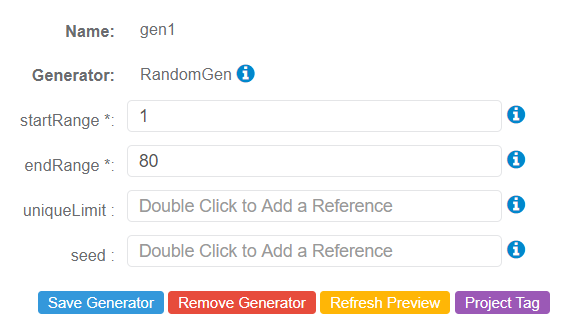### regularPay Attribute - Generator Configuration

The RegularPayGen Generator configures the regular pay based on the hours worked. For this configuration, the hours Attribute is referenced by the hoursWorked Parameter.### overTime Pay Attribute - Generator Configuration

The OvertimePayGen Generator configures the overtime pay based on the number of hours worked over the standard 40-hour work week. For this configuration, the hours Attribute is referenced by the hoursWorked Parameter.### totalPay Attribute - Generator Configuration

The CalcGen Generator adds the regular pay and overtime pay values together to determine the person's total pay based on hours worked.

For this configuration, the following has been configured:

• equation = var1+var2
• numberFormat = 0.00
• var1 = References regularPay Attribute
• var2 = References overtimePay Attrtibute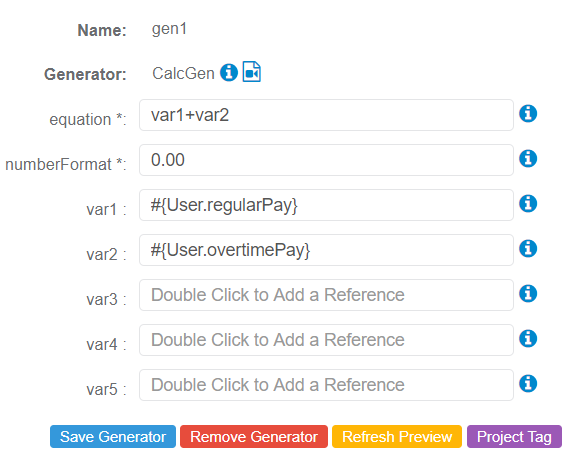## Video Training

The following video can be used to see a quick tutorial on how to use the CalcGen Generator: